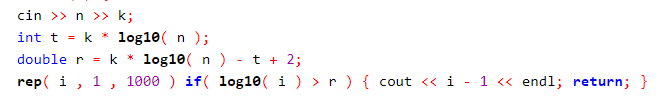10.23 & 10.24 写题

## 密码## 挑战

• 化简出来是 $A_j < A_i$， 这不可能，因为这意味着 $A_j > A_i > A_j + t_i , t_i > 0$
• $A_j > A_i + t_j$ ，也就是说不等式等价于 $A_j < A_j + t_i$ ，这个不等式必然成立。
• $A_i > A_j + t_i$ ，也就是说不等式等价于 $A_i + t_j < A_i$ ，这个不等式必然不成立。
• $A_i + t_j > A_j,A_j + t_i > A_i$ ，不等式就等价于 $A_i + t_j < A_j + t_i$ ，不难发现如果第二个条件成立，这个条件就一定成立，第三个条件成立，这个条件就一定不成立。所以说，对于 $A_i - t_i < A_j - t_j$ 的情况，我们把 $i$ 放在前面一定不劣于把 $j$ 放前面。对于这个东西不成立的情况，我们把 $i$ 放前面一定不优于 $j$ 放前面。